Next: A proposed public-key system Up: Subset-Sum (Knapsack) problems and Previous: Subset-Sum (Knapsack) problems and   Contents

## Subset-sum problems are hard

A subset of the numbers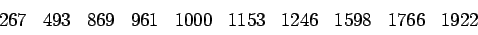adds up to 5842. Spend a few minutes trying to find such a subset. Whether you succeed or not, I hope you are convinced the task is not trivial.

This is an example of a subset-sum problem. In general, we are given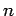natural numbersand a target numberand asked to find awith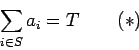A seemingly simpler problem is the subset-sum decision problem. For a givenand, decide whether there is anfor which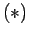holds, without being required to identify such an. However, it can be proved that the decision problem is just as difficult as the subset-sum problem in this sense:

Theorem 17   Suppose we had a method of solving the subset-sum decision problem. Then we could solve a subset-sum problem using the assumed methodtimes.

(theis not particularly important-- the main thing is that the number of uses of the method is not too large.)

Proof: Begin by using the method to decide ifis a sum of the-- if not, we can stop immediately. Then use the method to determine ifholds for some. If the answer is yes, we ignore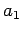for the rest of the analysis. If the answer is no, we know we must have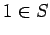. In this second case, we continue by using the method to decide whether there is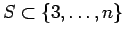with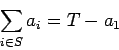A yes answer means we can assume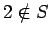, otherwise.

The idea of this proof is more important than the specific result. We show that one problem is as difficult as another by showing that a method of solving the supposedly easier problem can be used to solve another problem. This involves constructing one or several easier problems whose solution answers the hard problem. We saw another example of this idea in our discussion of the Rabin encryption system (section 3.3).

Using more elaborate versions of the techniques in Theorem 17, it can be shown that a method of solving the subset-sum decision problem could be used to solve many other problems, including:

• Factoring
• The Travelling Salesman Problem
• Any Integer Programming Problem
• The Satisfiability Problem
Don't worry if you are not familiar with the details of these problems. The important point is that they are all well-known problems for which many people have been unable to find efficient solution methods, which makes it unlikely that there is a method which solves all subset-sum decision problems efficiently (we will go into more detail on this in section 5).

The discussion above makes it plausible that some subset-sum problems are difficult. Further, there is some evidence that the typical'' subset-sum problem is not easy. V. Chvatal3 has shown that if theandare randomly chosen, then with high probablility (i) there will be nofor whichholds (ii) certain simple ways of proving this will not work.

#### Footnotes

... V. Chvatal3
Hard Knapsack Problems,'' Operations Research, vol. 28, pp 1402-1411Next: A proposed public-key system Up: Subset-Sum (Knapsack) problems and Previous: Subset-Sum (Knapsack) problems and   Contents
Translated from LaTeX by Scott Sutherland
2002-12-14